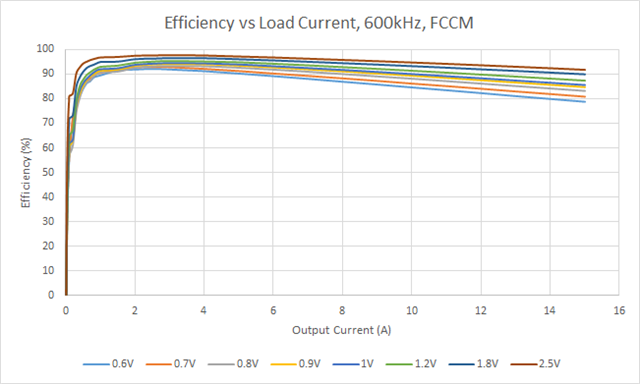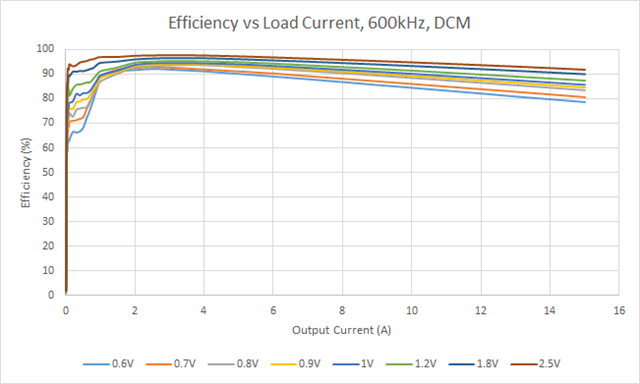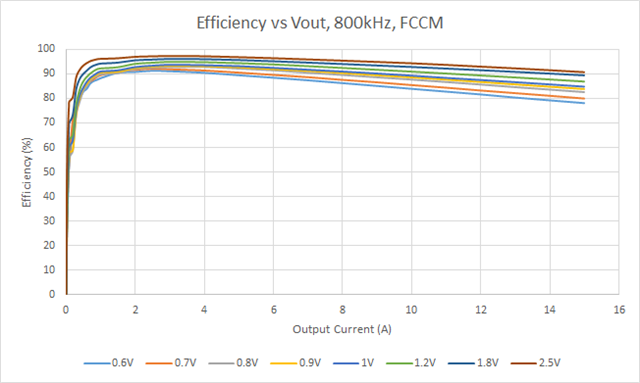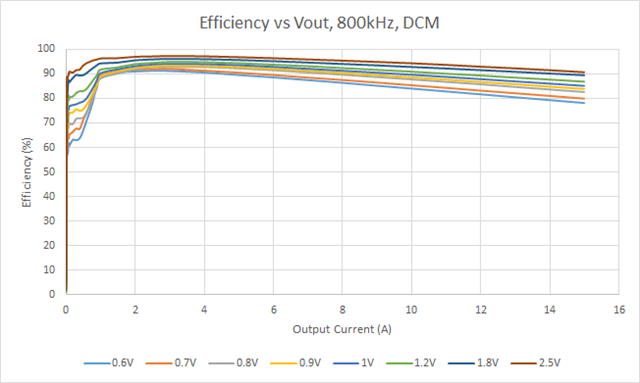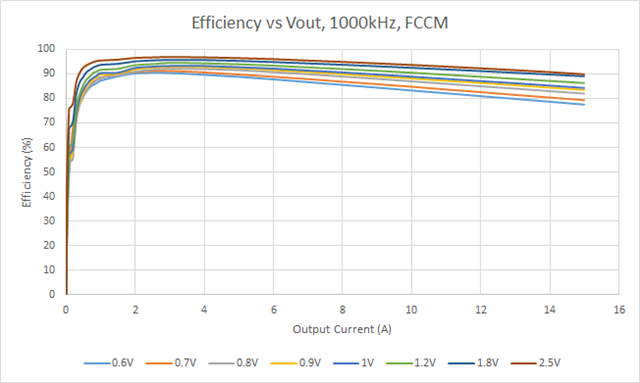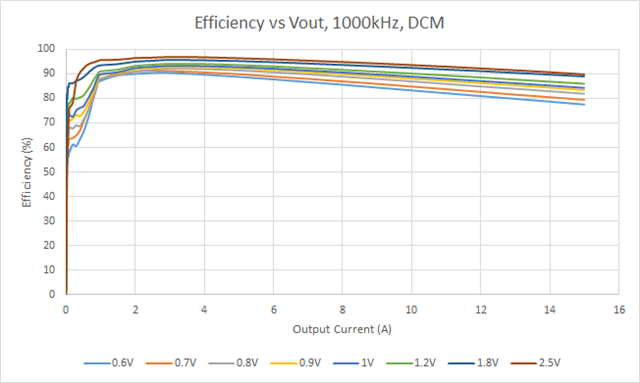If you have a related question, please click the "Ask a related question" button in the top right corner. The newly created question will be automatically linked to this question.

# [FAQ] TPS548A28: Efficiency & Output Voltage for 3.3V Input Voltage

Part Number: TPS548A28

How stable is the output voltage of the TPS548A28 when the input voltage is 3.3V and the output voltage is between 0.6V and 2.5V?

The stability of the output voltage of the TPS548A28 is relatively constant between 0.6V and 2.5V when the input voltage is 3.3V (with VCC and EN connected to VIN); the most notable difference is when the load current increases for 2.5V output voltage. The images below detail the output voltage for load currents ranging from 0A to 15A: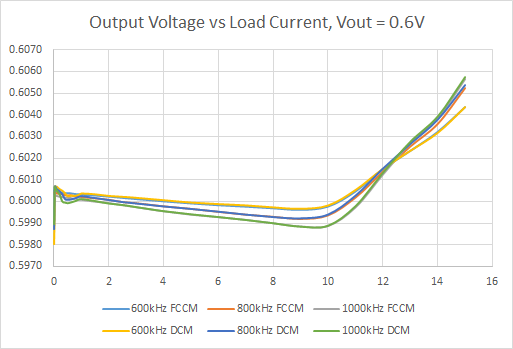At Vout = 0.6V, the output voltage is within 0.88% of the setpoint.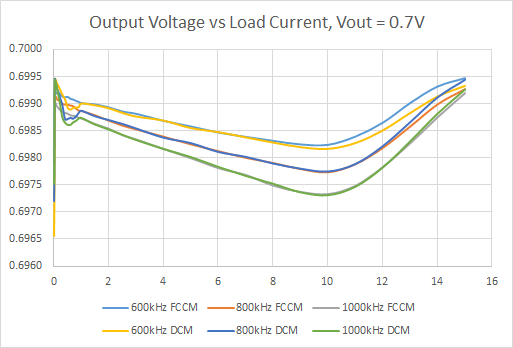At Vout = 0.7V, the output voltage is within 0.31% of the setpoint.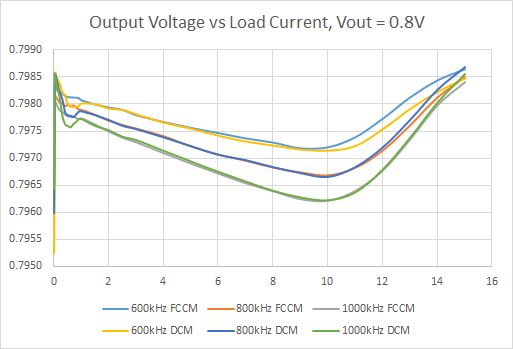At Vout = 0.8V, the output voltage is within 0.31% of the setpoint.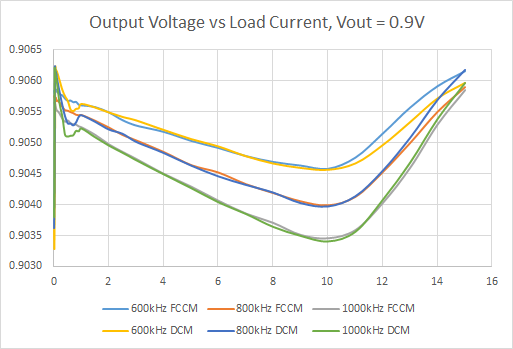At Vout = 0.9V, the output voltage is within 0.21% of the setpoint.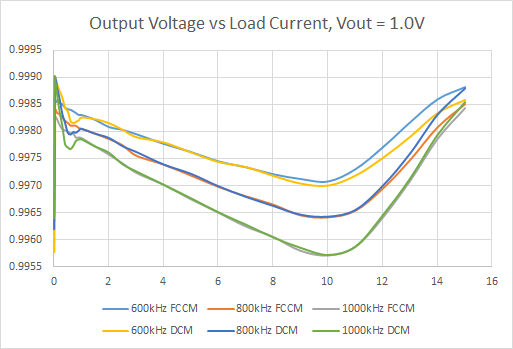At Vout = 1.0V, the output voltage is within 0.21% of the setpoint.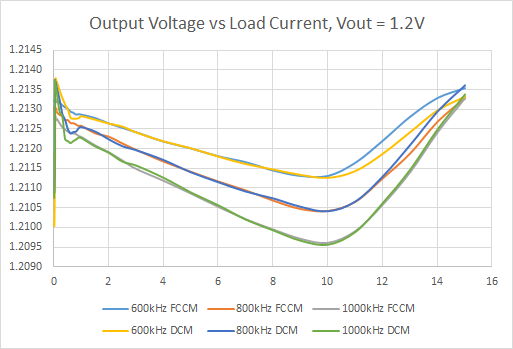At Vout = 1.2V, the output voltage is within 0.22% of the setpoint (the output voltage setpoint here is 1.212V due to resistor availability).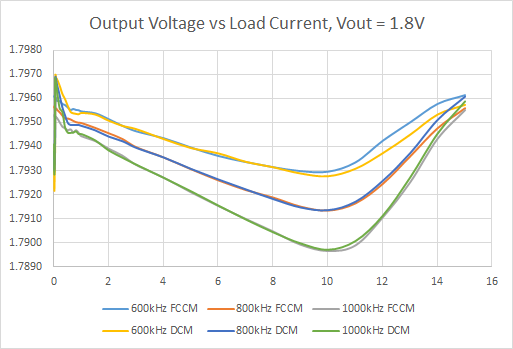At Vout = 1.8V, the output voltage is within 0.26% of the setpoint (the output voltage setpoint here is 1.794V due to resistor availability).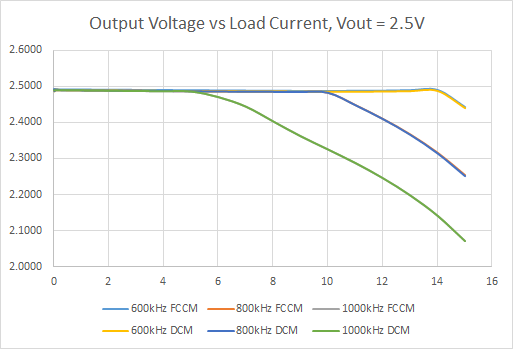At Vout = 2.5V, the output voltage is within 16% of the setpoint (explanation below).

The TPS548A28 has a minimum off-time fixed at 220ns. For a frequency of 1000kHz, this translates to a period of 1000ns with a maximum on-time of 780ns. Based on Section 8.2.3 Application Curves in the datasheet, the switching frequency increases with an increase in output voltage, and increases with a decrease in input voltage (it remains generally constant with load current). This means that, for a switching frequency setting of 1000kHz, the actual frequency will be greater than 1000kHz, meaning that the on-time will decrease substantially. Once the peak on-time has been reached, in order to maintain a constant output current, the output voltage begins to droop, as is seen in the above plot of the 2.5V output voltage.

As the switching frequency decreases (to 800kHz and 600kHz), the switching period, and as a result the maximum on-time, of the device increases, resulting in a greater current limit before the output voltage begins to droop. The droop begins at a load current of 14A, 10A, and 5A for switching frequencies 600kHz, 800kHz, and 1000kHz respectively.

What is the efficiency of the TPS548A28 over these output voltages with an input voltage of 3.3V and an output voltage between 0.6V and 2.5V?

The efficiency of the TPS548A28 is detailed in the below plots. As with the above measurements, the 3.3V is connected between VIN, VCC, and EN, with the load currents ranging from 0A to 15A: